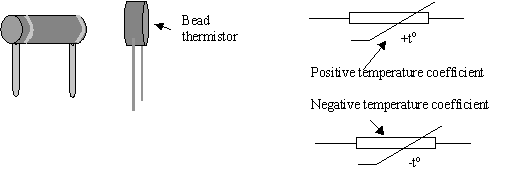# Topic 2 Logic Gates and Boolean AlgebraLogic gates are at the heart of digital electronics.  In digital electronics, we need to know nothing about electricity, other than the difference between on (1) and off (0).

Digital electronics is widely used in telecommunications, computers, and sound recording.

The NOT gate

The simplest of all the logic gates is the NOT gate.  Notice that when we write the name of a logic gate, we always write in UPPER CASE letters.  Truth tables summarise the output condition for a variety of input conditions.

For the NOT gate the truth table looks like this:

 INPUT OUTPUT 0 1 1 0

The AND gate

For the AND gate the truth table looks like this:

 A B OUTPUT 0 0 0 1 0 0 0 1 0 1 1 1

The OR gate

For the OR gate the truth table looks like this:

 A B OUTPUT 0 0 0 1 0 1 0 1 1 1 1 1

The Exclusive OR gate

For the EX-OR gate the truth table looks like this:

 A B OUTPUT 0 0 0 1 0 1 0 1 1 1 1 0

 The exclusive OR (EX-OR) will only return a high output is either one or the other input is high, but not both.

The NAND gate

For the NAND gate the truth table looks like this:

 A B OUTPUT 0 0 1 1 0 1 0 1 1 1 1 0

The NOR gate

For the NOR gate the truth table looks like this:

 A B OUTPUT 0 0 1 1 0 0 0 1 0 1 1 0

Now try Question 1

Combining Logic Gates

The output of one logic gate can be connected to the input of another.  The example is a simple system using a NAND gate with a NOT gate.

Try Question 2 to work out the output of the combination.  You should find that it’s the same as an AND gate

Now try something a little more complex:

Can you work out the output?  [Hint: label the output of each gate and work through logically.]  Then try Question 3.

Making Gates from NAND gates

It is more economical to make circuits from just one kind of chip and many circuits are made up of just NAND gates.  Let us look how:

The two inputs of a NAND gate connected together make the NAND gate into a NOT gate.

In this circuit below the output of a NAND gate is inverted by the NOT gate to produce the output of an AND gate.

We can show this in a truth table:

 A B C Q 0 0 1 0 0 1 1 0 1 0 1 0 1 1 0 1

Look at this circuit:

Answer Question 4 to show what the Output Q is

Boolean Algebra

Boolean algebra does the same job as a truth table, but with symbols.  A Boolean expression tells us what condition will give an output of 1.

For a NOT gate the Boolean expression is:

The symbol Ā is pronounced “A-bar”, and means that the state Q is opposite to the state Q.  So the statement says “Q is equal to NOT A”.  This means that the output Q is a 1 when A is a 0.  The truth table associated with the statement is:

 INPUT OUTPUT 0 1 1 0

For an AND gate the Boolean expression is:

The dot between the A and the B mean that both A AND B have to be 1 for Q to be 1.  The expression is pronounced, “Q equals A dot B”.  The equivalent truth table is:

 A B Q 0 0 0 1 0 0 0 1 0 1 1 1

For the OR gate the Boolean expression is:

This is pronounced, “Q is equal to A OR B” and the truth table is:

 A B Q 0 0 0 1 0 1 0 1 1 1 1 1

We looked at this example using truth tables.  Now we are going to analyse it using Boolean algebra.

Analyse this circuit using the questions.

Step 1 – Answer Question 5 to get the output Q.

Step 2 – Answer Question 6 to get the input D

Step 3 – Answer Question 7 to get the input C

Now you will need to look at some rules that will help you to simplify the expression you have just worked out:

 A.A = A                A + A = A               A.Ā = 0                A + Ā = 1    A + 1 = 1 = A.0 = 0                        A + 0 = A                  A = A (“NOT NOT A”)

Step 5 – Answer Question 9 to get Q in terms of A and B

The rules help us to simplify a lot of more complex expressions.  Have a go at Question 10 to test your understanding.

We can build a circuit using a Boolean expression, which we will look at now.

 A B OUTPUT 0 0 0 1 0 1 0 1 1 1 1 0

1. Look at where the output is 1.  It occurs when A is 1 and B is 0, we get a 1, orwhen A is 0 and B is 1.  We can write this in Boolean notation as:
 Q = A.B + A.B

1. This means that we need an OR gate to give us the output Q.  So here is the OR gate with its inputs:
2. To achieve the inputs we need to have two AND gates, each of which has ONE of its inputs inverted.  This part of the circuit will give out 1 when A = 0 and B = 1.
3. This part of the circuit will give out 1 when A = 1 and B = 0
4. So now we need to connect each part to the two inputs A and B.
5. Now we need to connect up our circuit to the OR gate: